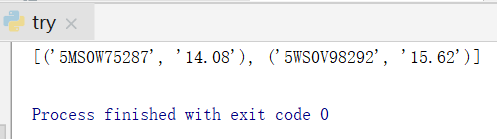``````import openpyxl
import xlrd

def get_infor():
book = xlrd.open_workbook('C:/Users/lenovo/Desktop/模板.xlsx')
sheet = book.sheet_by_name('WRT模板IN')
L =[]
for i in range (2,sheet.nrows):          # i 从第四列开始
PN = str(sheet.cell(i,5).value)
Cost_USD = str(sheet.cell(i, 9).value)
L.append((PN,Cost_USD))
#t=tuple(L)
return L
#print(L)

t1= get_infor()
#print(t1)
#
# sh = t1

# print(sh)
#
def Open_excel_cost():
sheet = workbook.worksheets   # 第一个页签
sheet['D2'] = t1
#sheet['A1'] = t1
workbook.save('C:/Users/lenovo/Desktop/文档模板/IN/Cost_IN.xlsx')  # 此步骤要保存才行的， 否则是不会在excel中显示的 2019.8.24
print(sheet['D2'].value)
print(sheet.title)

Open_excel_cost()

``````

1个回答Python如何将一个列表写入到一个excel表中的一列，求Python代码，谢啦！

Python如何将一个列表写入到一个excel表中的一列，求Python代码，谢啦！

Python读取excel个sheet，怎么将每个sheet的A列和B列数据放入单独list中。

python 匹配excel和txt文件，将匹配的关键词放在后面新建的一列

Python3中pandas批量汇总多个Excel文件，如何将中文日期命名的文件名变成数字日期并写入Excel第一行？

python 利用openpyxl 循环写入EXCEL 为什么只保留最后一条

1、python openpyxl 循环写入EXCEL 为什么只保留最后一条。在循环过程中每一行都其实都成功写入EXCEL文件，但循环到下一条都将上一条记录删除。最后只保留最后写入一条数据。 2、代码 ``` for href_url,roos in zip(href_url,href_name): addurl = href_url name = roos.xpath('./td/text()') i=i+1 #添加数据 #tree.insert("",i,text="",values=(i,name,addurl)) #得到企业详细信息 #url_id = "getxxgkContent&dataid=9339c790f8694f878b81e6fdc864be69" addurl=addurl[39:93] datas = {"xkgk": "getxxgkContent", "dataid": addurl} url = "http://permit.mee.gov.cn/permitExt/xkgkAction!xkgk.action?xkgk=" + addurl html = requests.get(url, headers=datas) soup = BeautifulSoup(html.text, 'lxml') name_id = soup.find_all('p', style="font-size:36px;").text # 得到企业名称 name_add = soup.find_all('p', style="font-weight: bold;color: green;font-size: 14px;").text # 得到企业地址等信息 ..strip() 属性删除空格 content=name_add content = content.strip() # 删除字符串左边空格 content = content.split() # 拆分字符串，通过指定分隔符对字符串进行分割，默认是空格。rstrip("\xa0\xa0\xa0\xa0\r\n\t\t\t") # content=content.partition(":") str2 = ''.join(content) u1, u2, u3, u4, u5 = str2.split('：', 4) f1 = u2.find('行业类别') f2 = u2[0:f1] g1 = u3.find('所在地区') g2 = u3[0:g1] h1 = u4.find('发证机关') h2 = u4[0:h1] ii=str(i) wb = Workbook() # 创建文件对象 # grab the active worksheet ws = wb.active # 获取第一个sheet cell = ws["A1"] cell.value = '序号' cell = ws['A'+ii] cell.value = i cell = ws["B1"] cell.value = '企业名称' cell = ws["B"+ii] cell.value = name cell = ws["C1"] cell.value = '生产经营场所地址' cell = ws["C"+ii] cell.value = f2 cell = ws["D1"] cell.value = '行业类别' cell = ws['D'+ii] cell.value = g2 cell = ws["E1"] cell.value = '所在地区' cell = ws['E'+ii] cell.value = h2 cell = ws["f1"] cell.value = '发证机关' cell = ws['f'+ii] cell.value = u5 wb.save("d:\\sample.xlsx") messagebox.showinfo("提示",ii) pass ``` #excel写入方式修改为，但最后只保留最后写入一条数据。 ``` wb = Workbook() # 创建文件对象 # grab the active worksheet ws = wb.active # 获取第一个sheet tableTitle = [a, b,c,] for col in range(len(tableTitle)): c = col + 1 ws.cell(row=1, column=c).value = tableTitle[col] # 数据表基本信息 tableValues = [[f2,g2, h2,]] for row in range(len(tableValues)): ws.append(tableValues[row]) # wb.save(ExcelFullName) wb.save("d:\\1.xlsx") pass ```

python 怎么实现一个excel表中，多个sheet中数据相加？

Python读取符合一定条件的Excel数据，复制到文本文件中

【python】怎样用python读取txt并按规则写入excle

python dataframe 用第一列数据修改index

Python 使用 openpyxl 将Excel数据 导入MySQL数据库中

Python 中如何将多行数据的文件转成一列数据的文件？

【python】用python读取txt并按规则写入excle

![图片说明](https://img-ask.csdn.net/upload/201608/09/1470722627_895105.jpg) 如图所示，在excel文件中，我想把第一个txt的数据写入excel的第一列，把第二个txt的数据写入excel的第二列中？现在我只能把两个txt文件的数据全部放在一列中，如何修改才能达到目的呢？码代如下： ``` #include <iostream> #include <fstream> #include <vector> #include <windows.h> #include <string> using namespace std; char* WcharToChar(const wchar_t* wp) { char *m_char; int len= WideCharToMultiByte(CP_ACP,0,wp,wcslen(wp),NULL,0,NULL,NULL); m_char=new char[len+1]; WideCharToMultiByte(CP_ACP,0,wp,wcslen(wp),m_char,len,NULL,NULL); m_char[len]='\0'; return m_char; } wchar_t* CharToWchar(const char* c) { wchar_t *m_wchar; int len = MultiByteToWideChar(CP_ACP,0,c,strlen(c),NULL,0); m_wchar=new wchar_t[len+1]; MultiByteToWideChar(CP_ACP,0,c,strlen(c),m_wchar,len); m_wchar[len]='\0'; return m_wchar; } wchar_t* StringToWchar(const string& s) { const char* p=s.c_str(); return CharToWchar(p); } int main() { const string fileform = "*.txt"; const string perfileReadPath = "Data"; const int perclass_sample = 20; string fileReadPath; int nLine = 0; string buf; vector<float> descriptors; int j = 0; ofstream file("data_file.csv"); fileReadPath = perfileReadPath + "/" + fileform; HANDLE hFile; LPCTSTR lpFileName = StringToWchar(fileReadPath); WIN32_FIND_DATA pNextInfo; hFile = FindFirstFile(lpFileName, &pNextInfo); if(hFile == INVALID_HANDLE_VALUE) { exit(-1); } do { if(pNextInfo.cFileName == '.') continue; j++; printf("%s\n",WcharToChar(pNextInfo.cFileName)); ifstream src_data(perfileReadPath + "/" + WcharToChar(pNextInfo.cFileName)); while( src_data ) { if( getline( src_data, buf ) ) { nLine++; descriptors.push_back( atof(buf.c_str()) ); } } src_data.close(); for(int i=0; i<descriptors.size();i++) { file<<descriptors[i]<<endl; } //格式化容器，清除容器内所有数据，为保存下一个txt文件数据文件做准备 descriptors.clear(); } while (FindNextFile(hFile,&pNextInfo) && j<perclass_sample); return 0; } ```

Python想要读取Excel中的两列数据，请问大家我这个有什么问题？

Python 3.6.4 (v3.6.4:d48eceb, Dec 19 2017, 06:54:40) [MSC v.1900 64 bit (AMD64)] on win32 Type "copyright", "credits" or "license()" for more information. >>> import numpy >>> from openpyxl import load_workbook ##读取路径 wb = load_workbook(filename=r'D:\密闭取芯综合数据.xlsx') ##读取名字为Sheet1的sheet表 ws = wb.get_sheet_by_name("密闭取芯综合数据") arr1 = [] arr2 = [] ## 遍历第2行到10000行 for row_A in range(2, 10000): ## 遍历第2行到10000行，第1列 a1 = ws.cell(row=row_A, column=23).value ## 遍历第2行到10000行，第3列 a2 = ws.cell(row=row_A, column=24).value if a1: # 写入数组1 arr1.append(a1) if a2: # 写入数组2 arr2.append(a2)

loonggg读完需要3分钟速读仅需 1 分钟大家好，我是你们的校长。我之前讲过，这年头，只要肯动脑，肯行动，程序员凭借自己的技术，赚钱的方式还是有很多种的。仅仅靠在公司出卖自己的劳动时...

MySQL数据库面试题（2020最新版）

!大部分程序员只会写3年代码

HTTP与HTTPS的区别